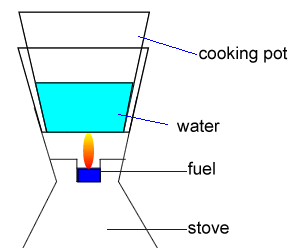Exercise from past exam. Ethanol (C2H5OH) is a common fuel burnt in some lightweight stoves suitable for camping, as shown on the right. Consider the following information. - Ethanol burns in excess air according to the following equation. C2H5OH(l) + 3O2(g) => 2CO2(g)  +  3H2O(g) /\  H= -1364 kJmol- -The cooking pot is made of aluminium and has a mass of 150g -The specific heat capacity of aluminium is 0.900Jg-1 oC-1 - The specific heat capacity of water is 4.18Jg-1 oC-1Calculate the minimum amount of energy, in kJ, required to heat 550g of water and the pot from 18.5oC to 100oC. SolutionCalculate the mass, in g, of ethanol that needs to be completely burnt to provide this energy. Solution Only 35% of the energy released by the combustion of ethanol is transferred to the cooking pot and contents. Calculate the mass, in g, of ethanol that needs to be burnt to heat the water and the pot from 18.5 oC to 100.0 oC. Solution Other camping stoves use butane (C4H10) as fuel. Given that, on complete combustion, 6.00 g of butane releases the same amount of energy as 10.0g of ethanol, calculate the magnitude of /\H, in kJ mol-, for the reaction below. 2C4H10(g) + 13O2(g) => 8CO2(g) + 10H2O(g) Solution